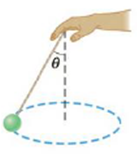Chapter 7, Problem 75AP

Chapter
Section
Textbook Problem

A 0.50-kg ball that is tied to the end of a 1.5-m light cord is revolved in a horizontal plane, with the cord making a 30° angle with the vertical. (See Fig. P7.75.) (a) Determine the ball’s speed. (b) If, instead, the ball is revolved so that its speed is 4.0 m/s, what angle does the cord make with the vertical? (c) If the cord can withstand a maximum tension of 9.8 N, what is the highest speed at which the ball can move?Figure P7.75

(a)

To determine
The speed of the ball.

Explanation

Given info: Mass of the ball (m) is 0.50 kg. Length of the rod (L) is 1.50 m.

Explanation:

The free body diagram is,

In the diagram, the vertical component of tension (T) is balanced by the weight of the ball. The horizontal component of tension equals the centripetal force.

Tcosθ=mgTsinθ=mv2r

• v is the speed of the ball.
• r is the distance between the ball and the center.
• g is the acceleration due to gravity

The distance between the ball and the center is,

r=Lsinθ

Therefore,

Tsinθ=mv2Lsinθ

From the above equations,

v=gLsin2θcosθ

Substitute 9

(b)

To determine
The angle of inclination when the speed of the ball is 4.0 m/s.

(c)

To determine
The highest speed at which the ball can move.

Still sussing out bartleby?

Check out a sample textbook solution.

See a sample solution

The Solution to Your Study Problems

Bartleby provides explanations to thousands of textbook problems written by our experts, many with advanced degrees!

Get Started

Find more solutions based on key concepts